edHelper subscribers - Create a new printable

Sample edHelper.com - LinearEquations Worksheet

 Name _____________________________Date ___________________
Linear Equations
Match the equation with its graph.
 8x + 3y2
=  7
 15
y  -
 395
= x
 x = -2 y - 10
 x = 7 + 1 y
23 =
 -11524
y  +
 2312
x
-2x = 34 + 6y

 1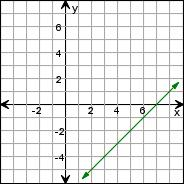2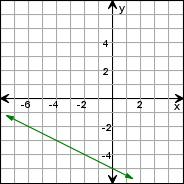3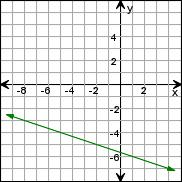4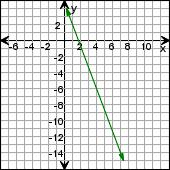5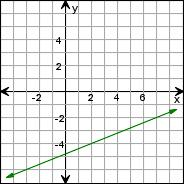6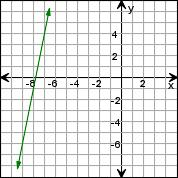Sample
This is only a sample worksheet.

edHelper subscribers - Create a new printable